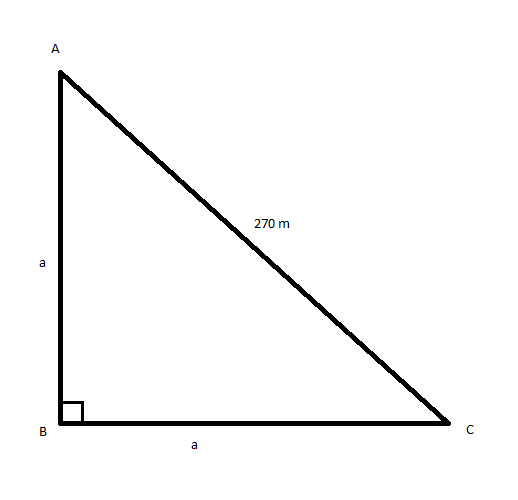QUESTION

# The area of a right-angled isosceles triangle, whose hypotenuse is equal to 270 m, is:A. $19,000{\text{ }}{{\text{m}}^2}$B. $18,225{\text{ }}{{\text{m}}^2}$C. $17,256{\text{ }}{{\text{m}}^2}$D. $18,325{\text{ }}{{\text{m}}^2}$

Hint: The area of a right-angled triangle is given by $\dfrac{1}{2} \times {\text{Height}} \times {\text{Base}}$. Pythagoras theorem states that ${\left( {{\text{Hypotenuse}}} \right)^2} = {\left( {{\text{Height}}} \right)^2} + {\left( {{\text{Base}}} \right)^2}$. In a right-angled isosceles triangle, the two adjacent sides at the right angle are equal in length.
Use this concept to solve this particular problem.

Let $\Delta ABC$ be right angled isosceles triangle with $\angle ABC = {90^0}$ and $AB = BC = a$Given hypotenuse $AC = 270{\text{ m}}$
By using Pythagoras theorem i.e., ${\left( {{\text{Hypotenuse}}} \right)^2} = {\left( {{\text{Height}}} \right)^2} + {\left( {{\text{Base}}} \right)^2}$ we have
$\Rightarrow {\left( {AC} \right)^2} = {\left( {AB} \right)^2} + {\left( {BC} \right)^2} \\ \Rightarrow \left( {270} \right) = {a^2} + {a^2} \\ \Rightarrow {\left( {270} \right)^2} = 2{a^2} \\ \Rightarrow {a^2} = \dfrac{{270 \times 270}}{2}{\text{ }}{{\text{m}}^2}.......................................\left( 1 \right) \\$
We know that the area of a right-angled triangle is given by $\dfrac{1}{2} \times {\text{Height}} \times {\text{Base}}$
So, the area of $\Delta ABC$ is
$\Rightarrow \Delta = \dfrac{1}{2} \times a \times a = \dfrac{{{a^2}}}{2}$
$\Rightarrow \Delta = \dfrac{{{a^2}}}{2} \\ \Rightarrow \Delta = \dfrac{{\dfrac{{270 \times 270}}{2}}}{2} \\ \Rightarrow \Delta = \dfrac{{270 \times 270}}{{2 \times 2}} \\ \therefore \Delta = 18,225{\text{ }}{{\text{m}}^2} \\$
Thus, the correct option is B. $18,225{\text{ }}{{\text{m}}^2}$